# Rutherford Island Width Evolution Equation

Suppose that the rigid wall surrounding the plasma is perfectly conducting (i.e.,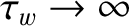, where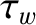is the time constant of the wall—see Section 3.10), and that there is no current flowing through the external magnetic field-coil [i.e.,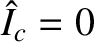, where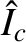is the normalized coil current—see Equation (3.194)]. In this case, Equations (3.187), (3.188), and (7.5), yield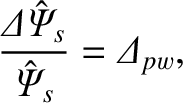(9.2)

where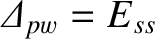is the (real dimensionless) perfect-wall tearing stability index,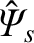the normalized reconnected helical magnetic flux at the rational surface [see Equation (3.184)], andthe normalized helical sheet current density at the rational surface [see Equation (3.183)]. It is clear from the previous equation that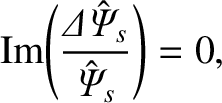(9.3)

which implies that zero electromagnetic torque is exerted at the rational surface. (See Section 3.13.)

Equation (9.2) yields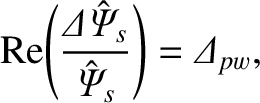(9.4)

which can be combined with Equations (8.1), (8.108), and (9.3) to give the so-called Rutherford island width evolution equation :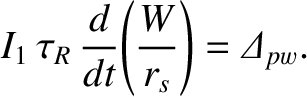(9.5)

Here,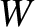is the full radial width of the magnetic island chain that develops at the rational surface,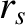the minor radius of the surface, and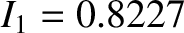[see Equation (8.124)]. We conclude that, in the nonlinear regime, the width of the magnetic island chain grows algebraically in time on the resistive diffusion time,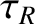. Obviously, this is a much slower time evolution than that predicted in the linear regime.